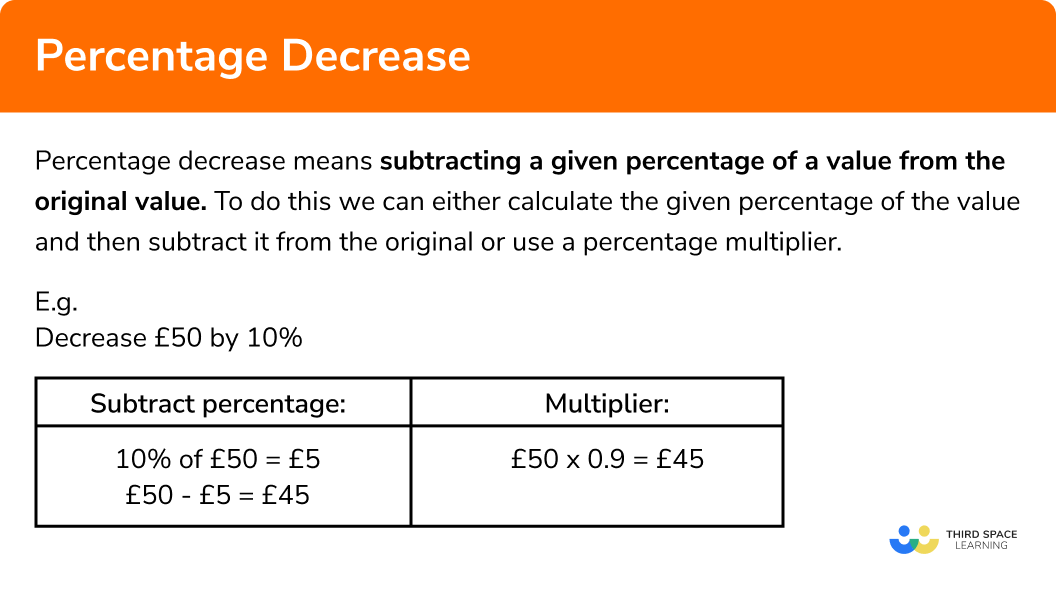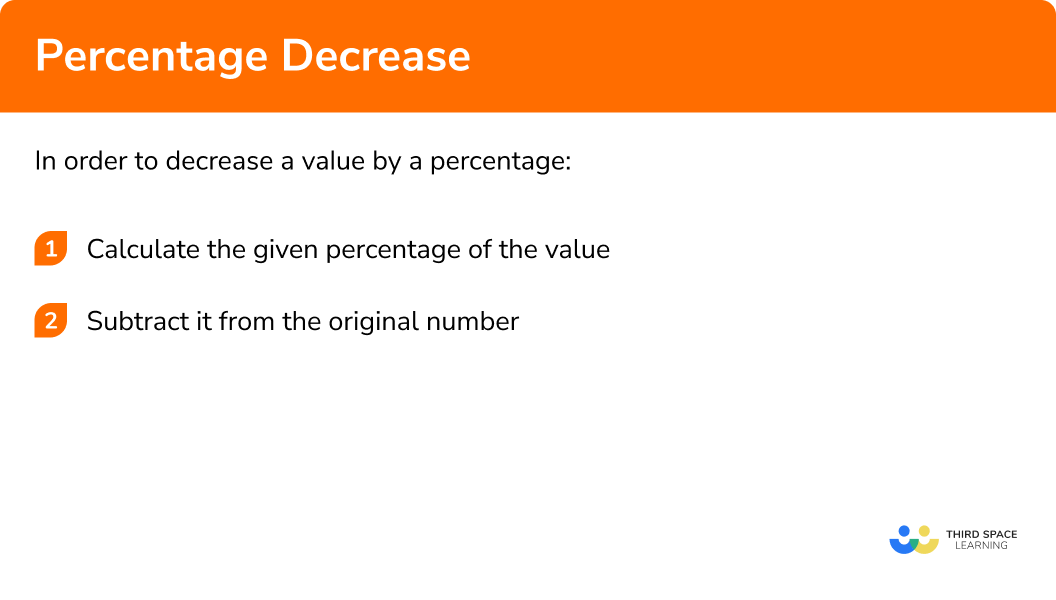GCSE Maths Number FDP Percentages

Percentage Decrease

# Percentage Decrease

Here we will learn about percentage decrease including how to decrease a value by a given percentage,  how to use multipliers to calculate percentage decrease and how to work out percentage change.

There are also percentage increase and decrease worksheets based on Edexcel, AQA and OCR exam questions, along with further guidance on where to go next if you’re still stuck.

## What is percentage decrease?

Percentage decrease means subtracting a given percentage of a value from the original value. To do this we can either calculate the given percentage of the value and then subtract it from the original or use a percentage multiplier.

### What is percentage decrease?## How to decrease a value by a percentage

In order to decrease a value by a percentage:

1. Calculate the given percentage of the value
2. Subtract it from the original number

### Explain how to decrease a value by a percentage in 2 steps## Percentage decrease examples

### Example 1: non-calculator

Decrease £80 by 30%

1. Calculate 30% of £80

To do this without a calculator, the easiest way is to calculate 10% (by dividing by 10) and then multiply by 3 to get 30%.

10% of £80 = £8

30% of £80 = £24

2 Subtract it from the initial value

£80 – £24 = £56

### Example 2: non-calculator

Decrease 250km by 45%

Calculate 45% of 250km

Subtract it from the original value

### Example 3: calculator

Decrease 760 by 39%

Calculate 39% of 760

Subtract it from the original value

### Example 4: calculator

A jumper costing £13.20 is reduced by 15% in a sale. Calculate the new price of the jumper.

Calculate 15% of £13.20

Subtract it from the original price

## How to decrease a value by a percentage using a percentage multiplier

We can decrease a value by a percentage using a percentage multiplier. A percentage multiplier is a decimal that is related to the percentage you are trying to find.

1. Subtract the percentage we are decreasing by from 100%

We subtract it from 100% because we are decreasing the value and 100% represents the original value.

2Convert to a decimal

3Multiply the original amount by the decimal

### Example 5: using a multiplier

Decrease 84m by 46%

Here we are calculating a 46 percent decrease so subtract 46% from 100%

Convert to a decimal. To do this we divide the percentage by 100.

Multiply the starting value by the decimal

### Example 6: using a multiplier

Daniel has £3600. He spends 27.5% of his money. How much does he have left?

This is a 27.5 percent decrease so subtract 27.5% from 100%

Convert to a decimal.

Multiply the value by the decimal

## Calculating percentage decrease

Given two values, we can calculate the percentage difference. This can also be called percentage decrease or percentage loss.

We can calculate percentage change using the percentage change formula:

$\text { Percentage change }=\frac{\text { Change }}{\text { Original }} \times 100$

The same formula can be used to calculate percentage increase.

1. Work out how much the value has changed by subtracting the final value from the original value
2. Apply the percentage change formula

### Example 7: calculating percentage change

Ricky weighed 70kg in March. By June his weight had decreased to 64.4kg. Calculate the percentage decrease in his weight.

His weight has changed from 70kg to 64.4kg. Work out the change:

Apply the percentage change formula. The change is 5.6kg and the original amount is 70kg.

### Example 8: calculating percentage loss

Louise buys a car for £7500 and sells it for £6150. Calculate Louise’s percentage loss.

The value has changed from £7500 to £6150. Work out the change:

Apply the percentage change formula. The change is £1350 and the original amount is £7500.

Percentage decrease is part of our series of lessons to support revision on percentages. You may find it helpful to start with the main percentages lesson for a summary of what to expect, or use the step by step guides below for further detail on individual topics. Other lessons in this series include:

### Common misconceptions

• Converting between percentages and decimals

Incorrectly converting percentages to decimals. The most common mistakes are with single digit percentages (e.g. 5%), multiples of 10 (e.g. 40%) and decimal percentage (e.g. 3.2%)

Remember to divide the percentage by 100 to find the decimal.

E.g.

$5 \%=0.05,\\ 40 \%=0.4,\\ 3.2 \%=0.032,\\ 106.5 \%=1.065$

• Using an incorrect value for the denominator in the percentage decrease formula

Using the new value instead of the original value for the denominator when calculating percentage change

### Practice percentage decrease questions

1. Decrease 7500m by 20\%

7480m7520m9000m6000m20\%   of  7500m is 1500m .

As this is a decrease we subtract from the original amount.

7500 – 1500 = 6000

2. Decrease 44ml by 82\%

7.92ml36.08ml80.08ml51.92ml82\% of 44ml is 36.08ml .

As this is a decrease we subtract from the original amount.

44 – 36.08 = 7.92

3. Use a multiplier to decrease £754 by 23\%

£580.58£731£173.42927.42The multiplier for this decrease is 0.77 , so the correct calculation is 0.77\times754

4.  Use a multiplier to decrease 254g by 38.2\%

215.8g6.65g97.028g156.972gThe multiplier for this decrease is 0.618 , so the correct calculation 0.618\times254

5. Find the percentage decrease when 650kg is decreased to 320kg

330\%50.77\%49.23\%203.125\%The actual decrease is 330kg .

To calculate the percentage decrease we divide this by the original amount and multiply by  100

\frac{650-320}{650}\times100

6. Find the percentage loss when Tristan buys a tractor for £11500 and sells the tractor for £9775

17.25\%85\%15\%82.25\%The actual loss is £1725 .

To calculate the percentage loss we divide this by the original amount and multiply by 100 .

\frac{11500-9775}{11500}\times100

### Percentage decrease GCSE questions

1. (a) A pair of trainers costing £35 are in a 25\% off sale. Calculate the sale price of the trainers.

(b) During the last week of the sale the trainers are reduced to £21 . Calculate the percentage decrease from the original price.

(4 marks)

(a)

25\% of £35 = £8.75

(1)

£35 – £8.75 = £26.25

(1)

(b)

£35 – £21 = £14

(1)

\frac{14}{35} \times 100=40\%

(1)

2. In 2018 a supermarket chain produced 308900 tonnes of packaging for its products. In 2019 they reduced the amount of packaging used by 4.7%

Calculate the amount of packaging used in 2019 .

(2 marks)

4.7\%   of  308900 = 14518.3

(1)

308900-14518.3 = 294381.7 tonnes

(1)

3. Samira wants to book a holiday for herself, her husband and their one child. There are two companies that she can book the holiday with. The prices of the holiday are shown below.

Travel Stars

£ 200 per child

£ 100 per child

10 % discount on early bookings

Samira is going to make an early booking. With which company would she get a better deal?

(5 marks)

Travel Stars: £400+£400+200 = £1000

(1)

Ready Jet Go: £500+£500+£100 = £1100

(1)

10\% of £1100 = £110

(1)

£1100-£110 = £990

(1)

Better deal with Ready Jet Go

(1)

## Learning checklist

You have now learned how to:

• Decrease a value by a given percentage
• Use a percentage multiplier to decrease a value by a percentage
• Calculate percent decrease between two values

## Still stuck?

Prepare your KS4 students for maths GCSEs success with Third Space Learning. Weekly online one to one GCSE maths revision lessons delivered by expert maths tutors.

Find out more about our GCSE maths tuition programme.